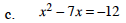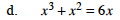### Home > PC > Chapter 1 > Lesson 1.2.1 > Problem1-72

1-72.
1.Solve the following equations. Homework Help ✎

1. 3(x − 2) − 2(x + 7) = 2x + 17

2. x2 + 3x − 10 = 0

3. x2 − 7x = − 12

4. x3 + x2 = 6x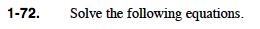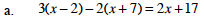What kind of equation is this?

It is linear. Distribute and solve for x.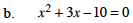What kind of equation is this?

What are three techniques for solving for x?

1. Factor and use the Zero Product Property.